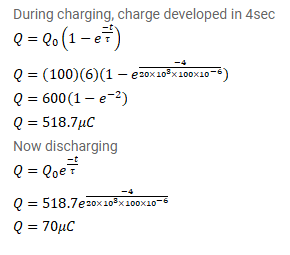# A capacitor of capacitance 100μF is connected across

Question:

A capacitor of capacitance $100 \mu \mathrm{F}$ is connected across a battery of emf $6.0 \mathrm{~V}$ through a resistance of $20 \mathrm{k} \Omega$ for $4.0 \mathrm{~s}$. The battery is then replaced by a thick wire. What will be the charge on the capacitor $4.0$ s after the battery is disconnected?

Solution: